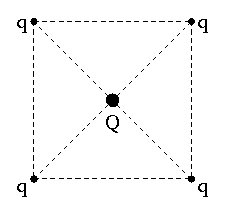# Electrostatics Equilibrium Problem.It is required to hold four equal point charges $+q$ each in equilibrium at the corners of a square. Find the charge in Coulombs, $Q$, that will do this if kept at the center of the square.


Details and Assumptions:

• $q=1\text{ C}.$
×